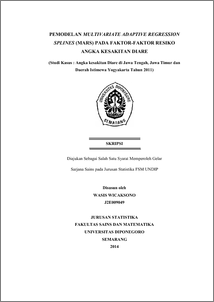# PEMODELAN MULTIVARIATE ADAPTIVE REGRESSION SPLINES (MARS) PADA FAKTOR-FAKTOR RESIKO ANGKA KESAKITAN DIARE (Studi Kasus : Angka kesakitan Diare di Jawa Tengah, Jawa Timur dan Daerah Istimewa Yogyakarta Tahun 2011)

WICAKSONO, WASIS (2014) PEMODELAN MULTIVARIATE ADAPTIVE REGRESSION SPLINES (MARS) PADA FAKTOR-FAKTOR RESIKO ANGKA KESAKITAN DIARE (Studi Kasus : Angka kesakitan Diare di Jawa Tengah, Jawa Timur dan Daerah Istimewa Yogyakarta Tahun 2011). Undergraduate thesis, FSM Undip.Preview
PDF
727Kb

## Abstract

Diarrhea morbidity can be interpreted as the number of patients with diarrhea in a region in the period of one year . Fluctuation in morbidity of diarrhea can be caused by environmental factors , educational factors , socio-economic factors , as well as nutritional and dietary factors . To reduce the number of spread of diarrhea needs to be done on the analysis of these factors are amenable to preventive measures against diarrheal diseases . To explain the relationship between the response variable and the predictor variables can be used by regression curve approach . Regression curve approach that is often used is the parametric regression approach , which assumed the form of the regression curve is known ( such as linear, quadratic, cubic) . If the parametric model assumptions are not met then the regression curve can be predicted using nonparametric regression model approach . Multivariate Adaptive Regression Spline ( MARS ) is a nonparametric regression method that can be used in data of high dimension . To get the best MARS models is done by combining Minimal Observation ( MO ) , Maximum Basis Function ( BF ) , and the Minimum Interaction ( MI ) by trial and error . MARS models were used to predict morbidity of diarrhea in Central Java , East Java and Yogyakarta is a model MARS ( MO = 2 ; BF = 28 ; MI = 3 ) in the form of the equation : Y = -0.526742 + 0.264444 * BF2 + 12.2382 * BF5 - 7.76719 * BF15 + 4.96445 * BF17 Keywords: Diarrhea Morbidity, Nonparametric regression, MARS

Item Type: Thesis (Undergraduate) H Social Sciences > HA Statistics Faculty of Science and Mathematics > Department of Statistics 43462 Mr Hasbi Yasin 12 Aug 2014 12:09 12 Aug 2014 12:09

Repository Staff Only: item control page